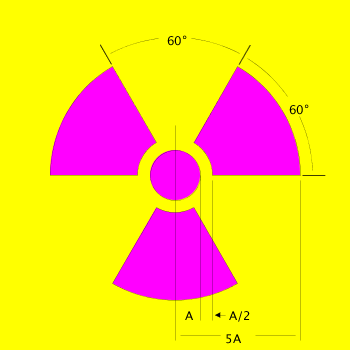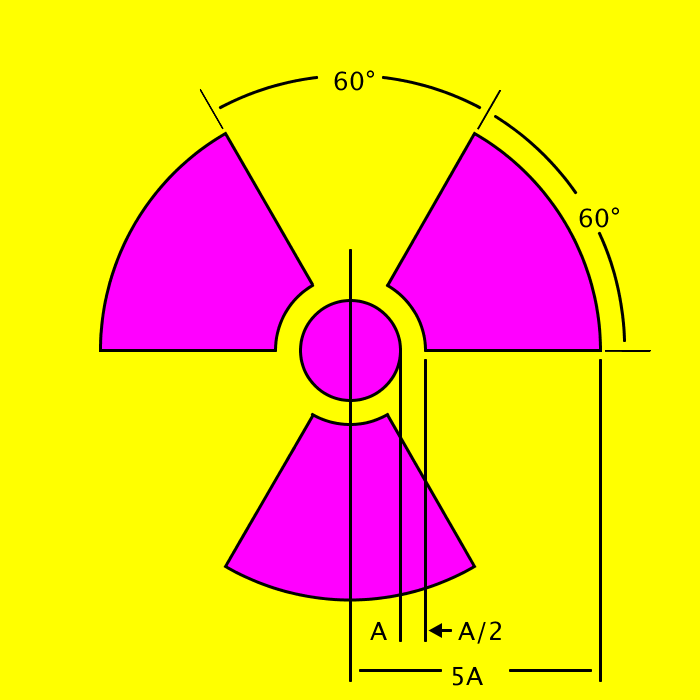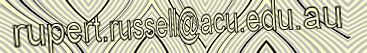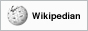Home > Tutorials > Processing > Processing Examples > Radiation Warning Sign Template#### processing code

```// Warning RadiationcSign
// I, the copyright holder of this work, release this work into the public domain. This applies worldwide.
// In some countries this may not be legally possible; if so:
// I grant anyone the right to use this work for any purpose, without any conditions, unless such conditions are required by law.
// by Rupert Russell
// http://www.rupert.id.au/tutorials/processing/examples.php
// Draws a Radiation warning sign based on the template at:
// https://www.osha.gov/OshStd_gif/10ZFZ_10.gif
// A local copy of this template is held on my website at: http://www.rupert.id.au/tutorials/processing/examples/radiation/images/10ZFZ_10.gif
// May 30 2014 v001

// The dimentions of this drawing are all based on the radius of the inner circle "A"

int A = 100;  // radius of inner circle defines the size of the sign and canvas and all artwork

int screenWidth = 7 * A;
int screenHeight = 7 * A;
float strokeWeightArc = A / 100 * 3;

color bgColor = color(255,255,255);
PFont font;

void setup() {
size(screenWidth,screenHeight);
ellipseMode(CENTER);
fill(255,0,255);  // Fill with Magenta
smooth();
noLoop();
}

void draw() {
background(255,255,0);

strokeWeight(A / 100);

// Define Variables for arcs
fill(255,0,255);  // Fill with Magenta
float a = screenWidth /2; // Center arc in canvas
float b = screenWidth /2; // Center arc in canvas
float c = 5 * A;
float d = 5 * A;
float start;
float stop;
ellipseMode(CENTER);  // Set ellipseMode to CENTER

// Draw  the line to divide the 2 outer arcs
a = screenWidth /2; // Center arc in canvas
b = screenWidth /2; // Center arc in canvas
fill(0);
stroke(0); // black

c = 6 * A;
d = 6 * A;
arc(a, b, c, d, start, stop, PIE );

// Draw the line to end  the 2 lower outer arc
a = screenWidth /2; // Center arc in canvas
b = screenWidth /2; // Center arc in canvas
fill(0);
stroke(0); // black
c = 6 * A;
d = 6 * A;
arc(a, b, c, d, start, stop, PIE );

// Draw the line to end  the 2 lower outer arc
a = screenWidth /2; // Center arc in canvas
b = screenHeight /2; // Center arc in canvas
fill(0);
stroke(0); // black
c = 6 * A;
d = 6 * A;
arc(a, b, c, d, start, stop, PIE );

// Draw outer yellow cirlce
// used to create a small gap between the ouside of the 3 main arcs
// and th dividing lines for the 60 degre markers
fill(255,255,0);  // Fill with yellow
noStroke();
ellipseMode(CENTER);  // Set ellipseMode to CENTER
ellipse(screenWidth /2, screenHeight /2, A * 5.1, A * 5.1);

// Draw arc 1
c = 5 * A;
d = 5 * A;
stroke(0);
strokeWeight(strokeWeightArc);
fill(255,0,255);  // Magenta
arc(a, b, c, d, start, stop, PIE );

// Draw arc 2
fill(255,0,255);  // Magenta
arc(a, b, c, d, start, stop, PIE );

// Draw arc 3
fill(255,0,255);  // Magenta
arc(a, b, c, d, start, stop, PIE );

// Draw middle cirlce
fill(255,255,0);  // Fill with yellow
noStroke();
ellipseMode(CENTER);  // Set ellipseMode to CENTER
ellipse(screenWidth /2, screenHeight /2, A + A/2, A + A/2);

// Draw inner cirlce
stroke(0);
fill(255,0,255);  // Fill with Magenta
ellipseMode(CENTER);  // Set ellipseMode to RADIUS
ellipse(screenWidth /2, screenHeight /2, A, A);

// Draw the inner outline of the 3 arcs
noFill();
stroke(0);
c = A + A/2;
d = A + A/2;

// Draw arc 1
arc(a, b, c, d, start, stop);

// Draw arc 2
arc(a, b, c, d, start, stop );

// Draw arc 3
arc(a, b, c, d, start, stop);

// Draw Outer arcs for 60 degree indicators

// Draw Outer arc 1 lower part
noFill();
stroke(0); // black line
strokeWeight(strokeWeightArc); // one step up from hairline default
c = (5 * A) + A / 2;
d = (5 * A) + A / 2;
arc(a, b, c, d, start, stop);

// Draw Outer arc 1 upper part
noFill();
stroke(0); // black line
strokeWeight(strokeWeightArc); // one step up from hairline default
c = (5 * A) + A / 2;
d = (5 * A) + A / 2;
arc(a, b, c, d, start, stop);

// Draw Outer arc 2 upper part
noFill();
strokeWeight(strokeWeightArc);
stroke(0);
c = (5 * A) + A / 2;
d = (5 * A) + A / 2;
arc(a, b, c, d, start, stop);

// Draw Outer arc 2 lower part
noFill();
strokeWeight(strokeWeightArc);
stroke(0);
c = (5 * A) + A / 2;
d = (5 * A) + A / 2;
arc(a, b, c, d, start, stop);

//draw the vertical center line for measuring the radius
strokeWeight(strokeWeightArc);
stroke(0);
float x1 = screenWidth /2;
float y1 = screenHeight /2 + A * 3.3;
float x2 = screenWidth /2;
float y2 = screenHeight /2 - A;
line(x1, y1, x2, y2);

//draw the vertical offset line for measuring the radius
strokeWeight(strokeWeightArc);
stroke(0);
x1 = screenWidth /2 + A/2;
y1 = screenHeight /2 + A * 2.9;
x2 = screenWidth /2 + A/2;
y2 = screenHeight /2;
line(x1, y1, x2, y2);

//draw the vertical offset line for measuring the width of the midle yellow circle
strokeWeight(strokeWeightArc);
stroke(0);
x1 = screenWidth /2 + A/2 + A/4;
y1 = screenHeight /2 + A * 2.9;
x2 = screenWidth /2 + A/2 + A/4;
y2 = screenHeight /2 + A / 10;
line(x1, y1, x2, y2);

//draw the offset line for measuring the width of 5A
strokeWeight(strokeWeightArc);
x1 = screenWidth /2 + A * 2.5;
y1 = screenHeight /2 + A * 3.3;
x2 = screenWidth /2 + A * 2.5;
y2 = screenHeight /2 + A / 10;
line(x1, y1, x2, y2);

// draw the horizontal line for the 5 A Gap left part
strokeWeight(strokeWeightArc);
stroke(0);

x1 = screenWidth /2 + A / 10;
y1 = screenHeight /2 + A * 3.2;
x2 = screenWidth /2 + A - A / 10;
y2 = screenHeight /2 + A * 3.2;
line(x1, y1, x2, y2);

// draw the horizontal line for the 5 A Gap right part
strokeWeight(strokeWeightArc);
stroke(0);
x1 = screenWidth /2 + A * 1.5  + A / 10;
y1 = screenHeight /2 + A * 3.2;
x2 = screenWidth /2 + (A * 2.5) - A / 10;
y2 = screenHeight /2 + A * 3.2;
line(x1, y1, x2, y2);

// draw the horizontal line for the A/2 pointer
strokeWeight(strokeWeightArc);
stroke(0);
x1 = screenWidth /2 + A/2 + A/4 + A/14;
y1 = screenHeight /2 + A * 2.8;
x2 = screenWidth /2 + A;
y2 = screenHeight /2 + A * 2.8;
line(x1, y1, x2, y2);

// draw the arrow head on the  horizontal line for the A/2 pointer
fill(0);
stroke(0,0,0);
// top pair of coordinates
x1 = screenWidth /2 + A * 0.9;
y1 = screenHeight /2 + A * 2.75;

// bottom pair of coordinates
x2 = screenWidth /2 + A * 0.9;
y2 = screenHeight /2 + A * 2.85;

// middle pair of coordinates
float x3 = screenWidth /2 + A/2 + A/4 + A/15;
float y3 = screenHeight /2 + A * 2.8;
triangle(x1, y1, x2, y2, x3, y3);
// triangle(30, 75, 58, 20, 86, 75);

// label the diagram
// calculate font size based on A
float tSize = A / 4;

// A
textSize(tSize);
fill(0);
x1 = screenWidth /2 + A /8;
y1 = screenHeight /2 +  A * 2.9;
text(" A", x1, y1);

// A/2
textSize(tSize);
fill(0);
x1 = screenWidth /2 + A ;
y1 = screenHeight /2 +  A * 2.9;
text(" A/2", x1, y1);

// 60 Alt 0176
x1 = screenWidth /2 - A / 4;
y1 = screenHeight /2 -  A * 2.6;
text(" 60°", x1, y1);

// 60 Alt 0176
x1 = screenWidth /2 + A * 2.2;
y1 = screenHeight /2 -  A * 1.23;
text(" 60°", x1, y1);

// 5A
textSize(tSize);
fill(0);
x1 = screenWidth /2 + A ;
y1 = screenHeight /2 + A * 3.35;
text("5A", x1, y1);

}```

http://www.onformative.com/codeformatter/

APA citation:
Russell, R. (2016, July 05, 07:26 am). Radiation Warning Sign Template.
Retrieved June 07, 2023, from http://www.rupert.id.au/tutorials/processing/examples/radiation/index.php

Last refreshed: June 07 2023. 04:49.50 pmThis work is licensed under a Creative Commons Attribution-NonCommercial-ShareAlike 2.5 License.

508 visits since May 30, 2014